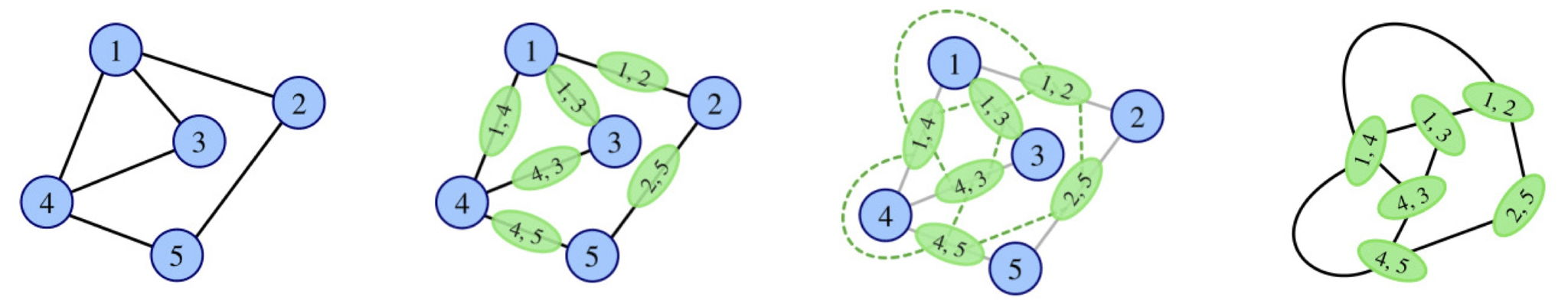시간 제한메모리 제한제출정답맞힌 사람정답 비율
5 초 512 MB169545.455%

## 문제

Last year, Rounddog participated in a contest with a pretty hard problem set and failed in solving the problem about line graphs. So recently he decided to study them deeper.

In the mathematical discipline of graph theory, the line graph of a simple undirected graph G is another simple undirected graph L(G) that represents the adjacency between every two edges in G. Precisely speaking, for an undirected graph G without loops or multiple edges, its line graph L(G) is a graph such that

• each vertex of L(G) represents an edge of G; and
• two vertices of L(G) are adjacent if and only if their corresponding edges share a common endpoint in G.Given a simple undirected graph G, Rounddog’s study aims to find the maximum cliques in its line graph L(G), and he decided to turn some of his findings into a challenge for you.

In this problem, you are given a simple undirected graph G and a small positive integer k. After finding all maximum cliques in Lk(G), where L0(G) = G and Ls(G) = L(Ls−1(G)) for each positive integer s, you need to tell Rounddog the number of vertices in a maximum clique in Lk(G), and also the number of distinct maximum cliques modulo 1 000 000 007.

Here, a subset of vertices of an undirected graph is called a clique if and only if there is an edge between each pair of vertices in the subset, and a maximum clique is a clique with the largest possible number of vertices.

## 입력

The input contains several test cases, and the first line contains a single integer T (1 ≤ T ≤ 1000), the number of test cases.

For each test case, the first line contains three integers n (1 ≤ n ≤ 100 000), m (0 ≤ m ≤ 200 000) and k (1 ≤ k ≤ 4): the number of vertices and edges in the given simple undirected graph G and the number of iterations of the line graph operation.

Then m lines follow, describing all edges of the graph. Each of them contains two integers u and v (1 ≤ u, v ≤ n, u ≠ v), representing an edge between vertices u and v.

It is guaranteed that the sum of n in all test cases does not exceed 2 000 000, the sum of m does not exceed 3 000 000, and the graph in each test case contains no loops and no multiple edges.

## 출력

For each test case, output a single line with two integers: first the number of vertices in a maximum clique, and then the number of distinct maximum cliques modulo 1 000 000 007.

## 예제 입력 1

3
5 0 4
5 4 1
1 2
1 3
1 4
1 5
5 4 4
1 2
1 3
1 4
1 5


## 예제 출력 1

0 1
4 1
6 12